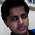### Car Problem

Source: www.quantstudy.com

Problem: Let A and B be two cities, with two different roads connecting them. Suppose that two cars can travel from A to B on different roads, keeping a distance that does not exceed 1 mile between them.  Is it possible for two cars to travel one from A to B, the other from B to A such that the distance between them is always greater than one mile?

Update: (23 January 2011)
Solution: Posted by Gaurav Sinha (chera) (CSE IITK 1996 Graduate, Now working at Indian Revenue Service) in comments! A more general solution with clear explanation posted by Ameya Ranade (Microsoft Software Engineer, CSE IITB 2009 Graduate)

1.its not possible. proof is by contradiction as follows:.

situation 1 : cars x,y move from A to B such that always d(x,y)<1.

situation 2: cars u,v move from A to B and B to A respectively s.t. always d(u,v)>1.

where x and u are on one road and y and v are on other road.

now, by appropriate scaling, choose the time trajectories of all cars in such a way that cars x and u start at same time from city A and are together subsequently. By the time it reaches city B, car y will meet car v at say time t when x and u are together and , y and v are also together.
At time t, 1< d(u,v)=d(x,y) < 1,

2.No.
Let P1, P2 be such that P1(t) is the location of car 1 on path 1 at time t. Similarly, P2(t) is the location of car 2 on path 2 at time t.
Now we know d(p1(t),p2(t))<1 mile for all t.
Let f be a fn. such that, f(p1(t)) = p2(t).

Now p2(t) takes all values from A to B.
Hence, its not possible

3.well if u want to see the borderline case then it is the following:

consider a circle of diameter 1 centered at the origin. City A is at (-1,0) and B is at (1,0). One road is from the top, the other from below. Consider equal constant speeds in both cases. This is the best we can do.

4.@chera.. nice solution
@shantanu.. I don't think I understand your solution. Please explain in more detail.

5.I have one solution. Let length of 2 roads be p and q. Draw a rectangle with sides p and q at origin and in +ve x and y. here any point (x,y) represent car1 at x distance on road1 and car2 at y distance on road2 at some time. Now, at A, we are at (0, 0) and at be at (p, q). Let dis(x,y) = actual distance between the cars. From given condition, there is a path between (0, 0) and (p,q) in rectangle such that, dis(x, y) <= 1 mile at every (x,y) in the path.

In the next case we are starting at (0, q) and ending at (p, 0). By simple path drawing we can see that this path will definitely intersect the last path at some point. Thus, the dustance <= 1 mile. Hence, proved that no such path can exist.

6.Correct solution! Thanks for the simple explanation.

7.solution of stainless is complete and correct.

solution posted by me is not complete because it is based on assumption that the 2 cars travelling on same road from A to B can travel together at all times. This is possible if there is no back gear and the cars only move forward. But its not clear to me whether this is possible in a general case, when the cars need to use back gear also.

8.@chera. thanks for the clarification. I agree with you.

9.chera, I guess there's another problem. We can find some x and y that move with d < 1, but it's not clear that for any x, there's such a y.